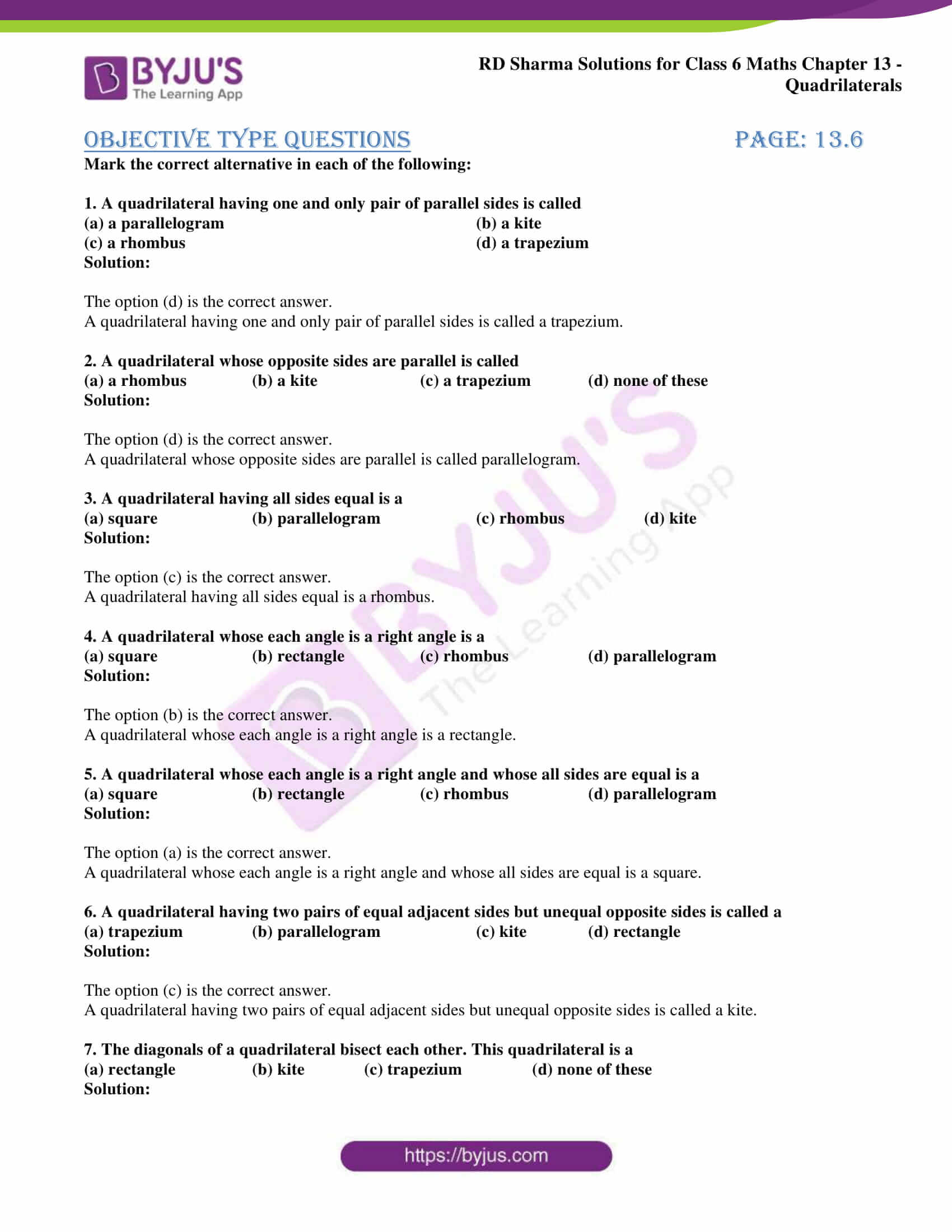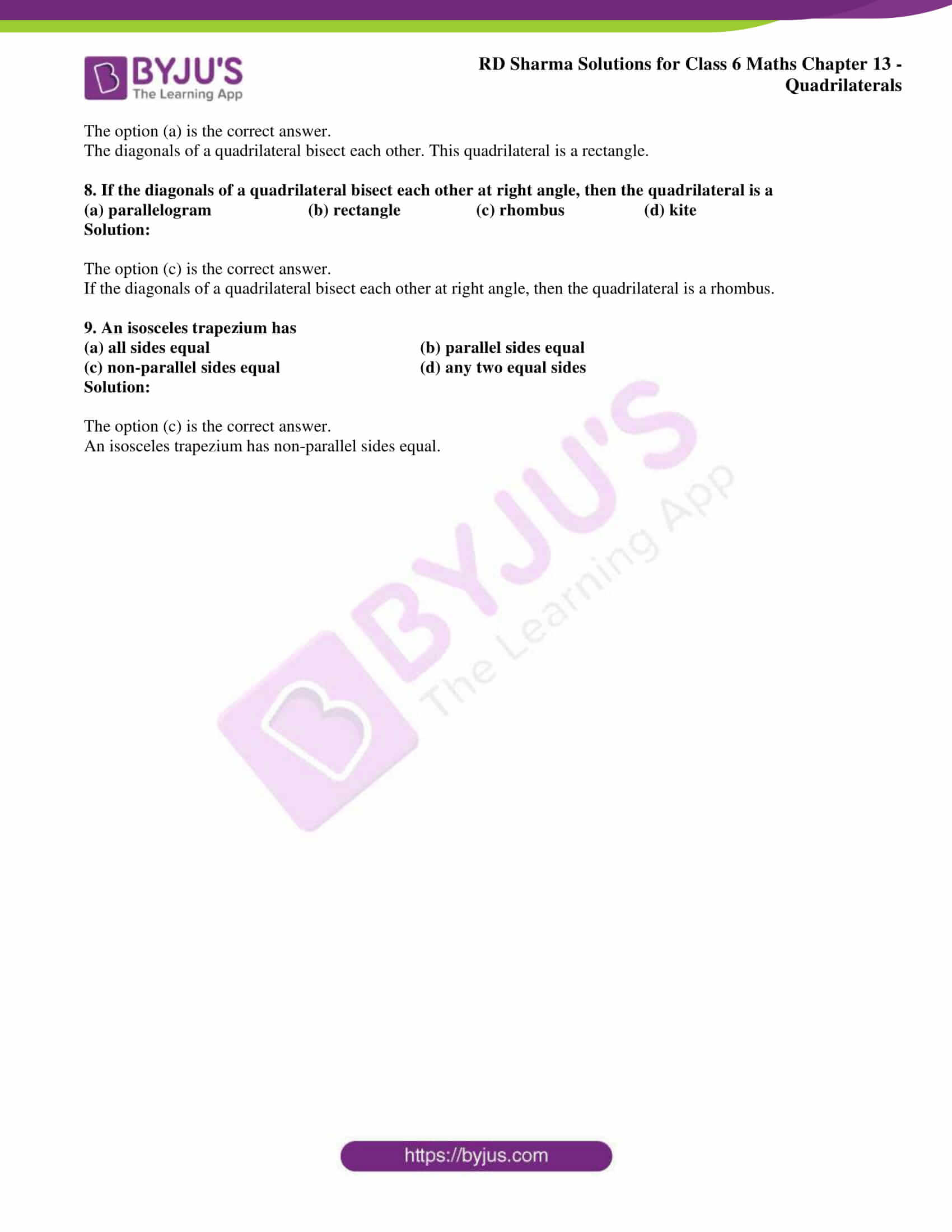# RD Sharma Solutions for Class 6 Maths Chapter 13: Quadrilaterals

The figure which is obtained by four line segments and three vertices are called Quadrilaterals. In this Chapter, the students get an idea about the types of Quadrilaterals and important formulas which are used to determine the sides and angles. The students can download PDF of either exercise or chapter-wise solutions which can be referred to clear doubts.

The solutions designed by faculty at BYJU’S helps students to improve their problem solving and logical thinking abilities. To know more about the concepts which are covered in this Chapter, the students can download RD Sharma Solutions for Class 6 Chapter 13 Quadrilaterals which are available here.## Access answers to Maths RD Sharma Solutions for Class 6 Chapter 13: Quadrilaterals

Objective Type Questions page: 13.6

Mark the correct alternative in each of the following:

1. A quadrilateral having one and only pair of parallel sides is called

(a) a parallelogram (b) a kite

(c) a rhombus (d) a trapezium

Solution:

The option (d) is the correct answer.

A quadrilateral having one and only pair of parallel sides is called a trapezium.

2. A quadrilateral whose opposite sides are parallel is called

(a) a rhombus (b) a kite (c) a trapezium (d) none of these

Solution:

The option (d) is the correct answer.

A quadrilateral whose opposite sides are parallel is called parallelogram.

3. A quadrilateral having all sides equal is a

(a) square (b) parallelogram (c) rhombus (d) kite

Solution:

The option (c) is the correct answer.

A quadrilateral having all sides equal is a rhombus.

4. A quadrilateral whose each angle is a right angle is a

(a) square (b) rectangle (c) rhombus (d) parallelogram

Solution:

The option (b) is the correct answer.

A quadrilateral whose each angle is a right angle is a rectangle.

5. A quadrilateral whose each angle is a right angle and whose all sides are equal is a

(a) square (b) rectangle (c) rhombus (d) parallelogram

Solution:

The option (a) is the correct answer.

A quadrilateral whose each angle is a right angle and whose all sides are equal is a square.

6. A quadrilateral having two pairs of equal adjacent sides but unequal opposite sides is called a

(a) trapezium (b) parallelogram (c) kite (d) rectangle

Solution:

The option (c) is the correct answer.

A quadrilateral having two pairs of equal adjacent sides but unequal opposite sides is called a kite.

7. The diagonals of a quadrilateral bisect each other. This quadrilateral is a

(a) rectangle (b) kite (c) trapezium (d) none of these

Solution:

The option (a) is the correct answer.

The diagonals of a quadrilateral bisect each other. This quadrilateral is a rectangle.

8. If the diagonals of a quadrilateral bisect each other at right angle, then the quadrilateral is a

(a) parallelogram (b) rectangle (c) rhombus (d) kite

Solution:

The option (c) is the correct answer.

If the diagonals of a quadrilateral bisect each other at right angle, then the quadrilateral is a rhombus.

9. An isosceles trapezium has

(a) all sides equal (b) parallel sides equal

(c) non-parallel sides equal (d) any two equal sides

Solution:

The option (c) is the correct answer.

An isosceles trapezium has non-parallel sides equal.